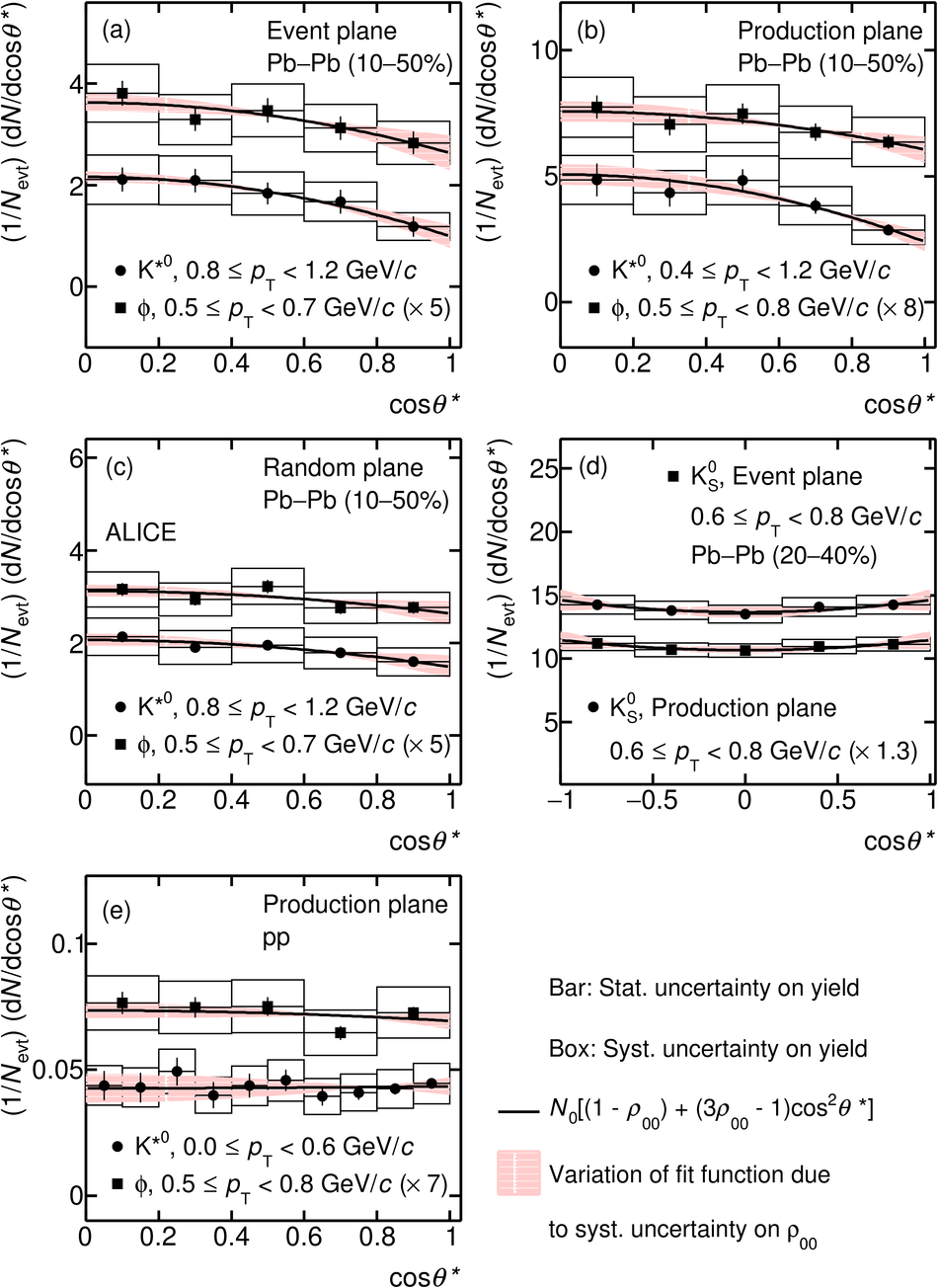# Measurement of spin-orbital angular momentum interactions in relativistic heavy-ion collisions

The first measurement of spin alignment of vector mesons ($\rm K^{*0}$ and $\phi$) in heavy-ion collisions at the Large Hadron Collider (LHC) is reported. The measurements are carried out as a function of transverse momentum ($p_{\rm T}$ ) and collision centrality with the ALICE detector using the particles produced at midrapidity ($|y| <~$ 0.5) in Pb-Pb collisions at a center-of-mass energy ($\sqrt{s_{\rm NN}}$) of 2.76 TeV. The second diagonal spin density matrix element ($\rho_{\rm 00}$ ) is measured from the angular distribution of the decay daughters of the vector meson in the decay rest frame, with respect to the normal of both the event plane and the production plane. The $\rho_{\rm 00}$ values are found to be less than 1/3 (= 1/3 implies no spin alignment) at low $p_{\rm T}$ ($<~$ 2 GeV/$c$) for both vector mesons. The observed deviations from 1/3 are maximal for mid-central collisions at a level of 3$\sigma$ for $\rm K^{*0}$ and 2$\sigma$ for $\phi$ mesons. As control measurements, the analysis is also performed using the $K_{\rm S}^{\rm 0}$ meson, which has zero spin, and for the vector mesons in pp collisions; in both cases no significant spin alignment is observed. The $\rho_{\rm 00}$ values at low $p_{\rm T}$ with respect to the production plane are closer to 1/3 than for the event plane; they are related to each other through correlations introduced by the elliptic flow in the system. The measured spin alignment is surprisingly large compared to the polarization measured for $\Lambda$ hyperons, but qualitatively consistent with the expectation from models which attribute the spin alignment to a polarization of quarks in the presence of large initial angular momentum in non-central heavy-ion collisions and a subsequent hadronization by the process of recombination.

Submitted to: PRL
e-Print: arXiv:1910.14408 | PDF | inSPIRE
CERN-EP-2019-251

Figures

## Figure 1

 Angular distribution of the decay daughter in the rest frame of the meson with respect to the quantization axis at $|y| <$ 0.5 for pp collisions at \s = 13 TeV and \pb collisions at \snn = 2.76 TeV. Panels (a) - (c) show results for \kst~and \ph with respect to EP, PP, and random event plane. Panels (d) and (e) are the results for \Kzs~with respect to both the PP and EP and for vector mesons in pp collisions with respect to PP, respectively## Figure 2

 Transverse momentum dependence of \rh{} corresponding to \kst, \ph, and \Kzs~mesons at $|y| <$ 0.5 in Pb--Pb collisions at \snn = 2.76 TeV and minimum bias pp collisions at \s = 13 TeV Results are shown for spin alignment with respect to event plane (panels a,b), production plane (c,d) and random event plane (e,f) for \kst~(left column) and \ph (right column) The statistical and systematic uncertainties are shown as bars and boxes, respectively.## Figure 3

 Measurements of \rh~as a function of $\langle N_{\mathrm {part}} \rangle$ for \kst~and \ph mesons at ranges of low and high \pt in \pb collisions. The statistical and systematic uncertainties are shown as bars and boxes, respectively. Few data points are shifted horizontally for better visibility.## Figure 4

 \rh~values from data in 10--50\% \pb collisions at 0.8 $<$ \pt $<$ 1.2 GeV/$c$ with respect to various planes compared with expectations from model simulations with and without added elliptic flow ($v_{2}$). The statistical and systematic uncertainties are shown as bars and boxes, respectively.